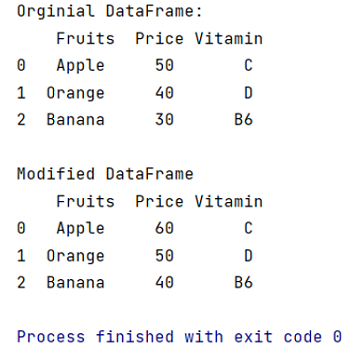# How to update a DataFrame in pandas while iterating row by row?

Learn how to update a DataFrame in pandas while iterating row by row?
Submitted by Pranit Sharma, on May 14, 2022

Rows in pandas are the different cell (column) values that are aligned horizontally and also provide uniformity. Each row can have the same or different value. Rows are generally marked with the index number but in pandas, we can also assign index names according to the needs.

In this article, we are going to learn how to update a DataFrame row while iterating? For this purpose, we are going to use pandas.DataFrame.iterrows() method.

## pandas.DataFrame.iterrows() method

This special function allows us to iterate each row in the DataFrame.

Syntax:

```DataFrame.iterrows()
```

To work with pandas, we need to import pandas package first, below is the syntax:

```import pandas as pd
```

Let us understand with the help of an example:

```# Importing pandas package
import pandas as pd

# Creating dictionary
d = {
'Fruits':['Apple','Orange','Banana'],
'Price':[50,40,30],
'Vitamin':['C','D','B6']
}

# Creating DataFrame
df = pd.DataFrame(d)

# Display original DataFrame
print("Original DataFrame:\n",df,"\n")

# Iterating rows and updating price
# of each item by +10
for i,row in df.iterrows():
price = 10
if row['Price'] <=60:
price += row['Price']
df._set_value(i,'Price',price)

# Display modified DataFrame
print("Modified DataFrame\n",df)
```

Output:Preparation